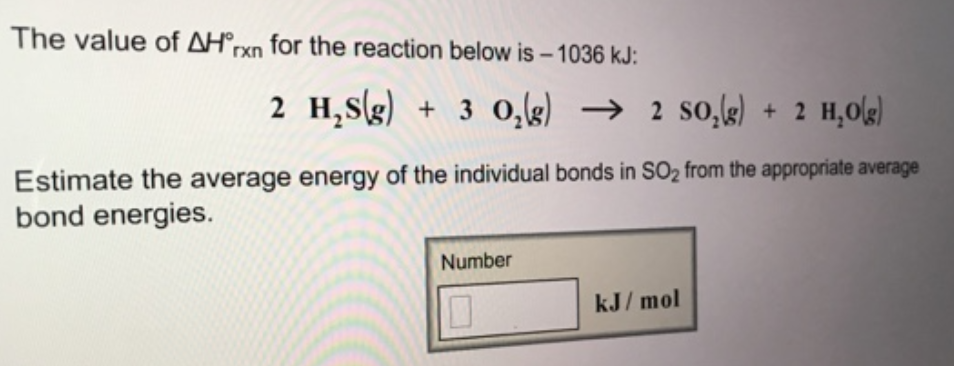# Problem: The value of ΔH°rxn for the reaction below is - 1036 kJ: 2H2S (g) + 3O2 (g) → 2SO2 (g) + 2H2O (g) Estimate the average energy of the individual bonds in SO2 from the appropriate average bond energies.

###### FREE Expert Solution
98% (273 ratings)###### Problem Details

The value of ΔH°rxn for the reaction below is - 1036 kJ:

2H2S (g) + 3O2 (g) → 2SO2 (g) + 2H2O (g)

Estimate the average energy of the individual bonds in SO2 from the appropriate average bond energies.